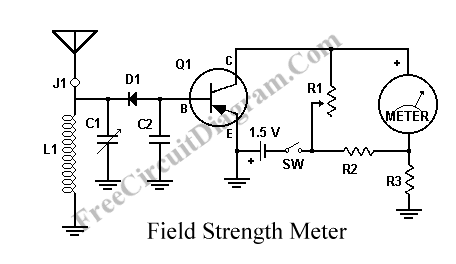# Another RF Field Strength MeterThis circuit is another radio frequency (rf) field strength meter, similar with our previous field strength meter circuit, but with lower supply voltage (only a single cell 1.5V battery). Here is the schematic diagram of this rf strength meter circuit:The parts list:

• Q1: 2N107 or GE-2, etc. (This is a pretty old circuit design. Any equivalent PNP transistor should work.)
• R1: 50K potentiometer
• R2: 1K, 1/2 watt
• R3: 1K, 1/2 watt
• J1: jack for antenna; the antenna must be insulated from the case
• D1: 1N34
• meter: milliammeter, 0 to 1 mA range
• antenna: a 30 to 50 centimeter piece of stiff wire, or a generic telescoping antenna
• L1 and C1 are chosen so that they form a resonant tank circuit at the frequency of interest.

[Circuit’s schematic diagram source: Meddium Wave Alliance]